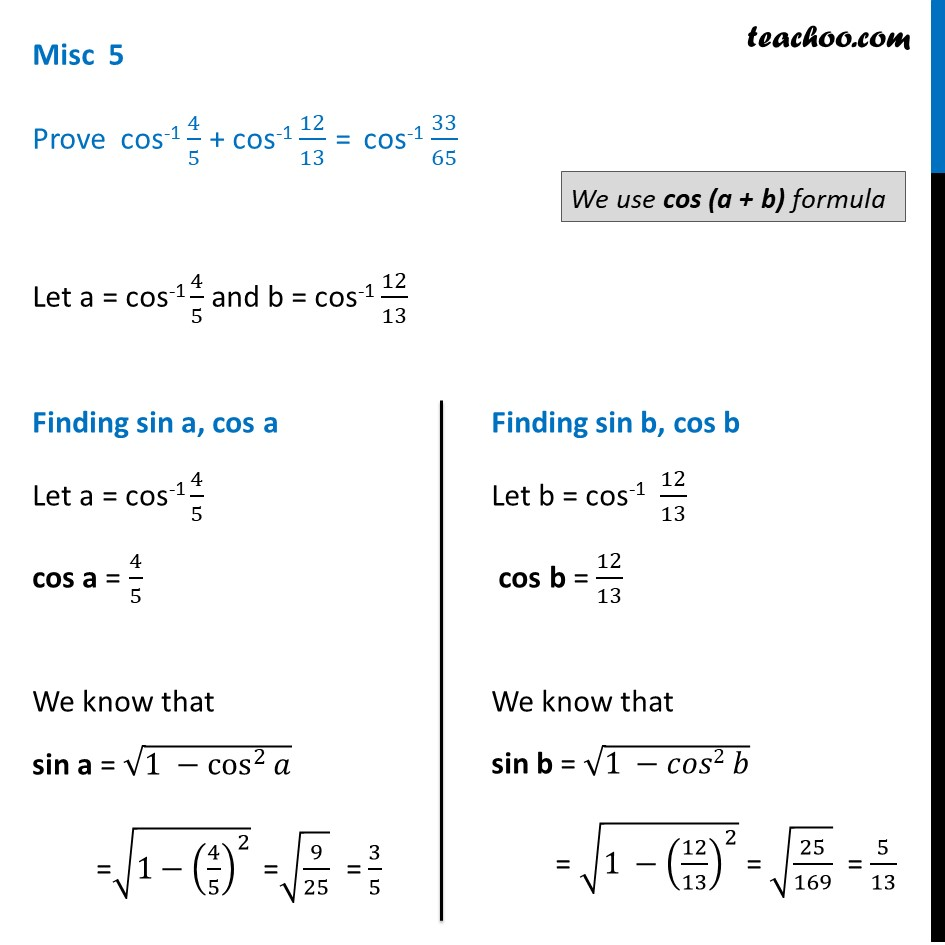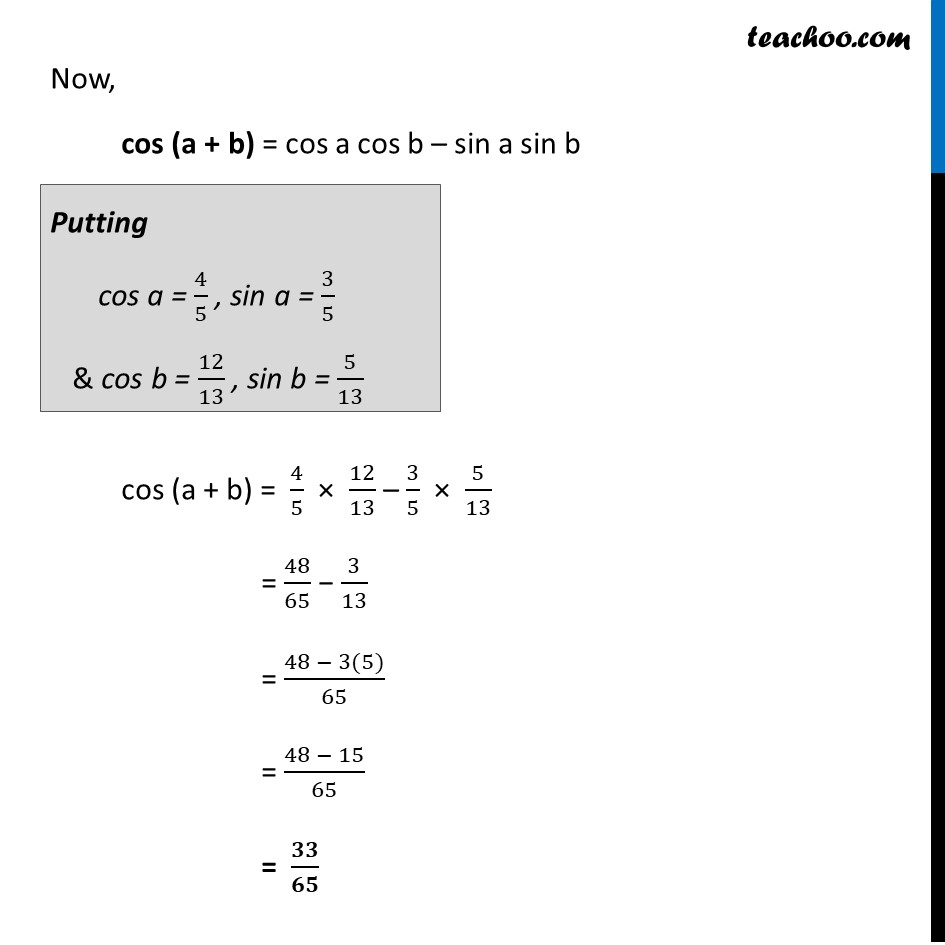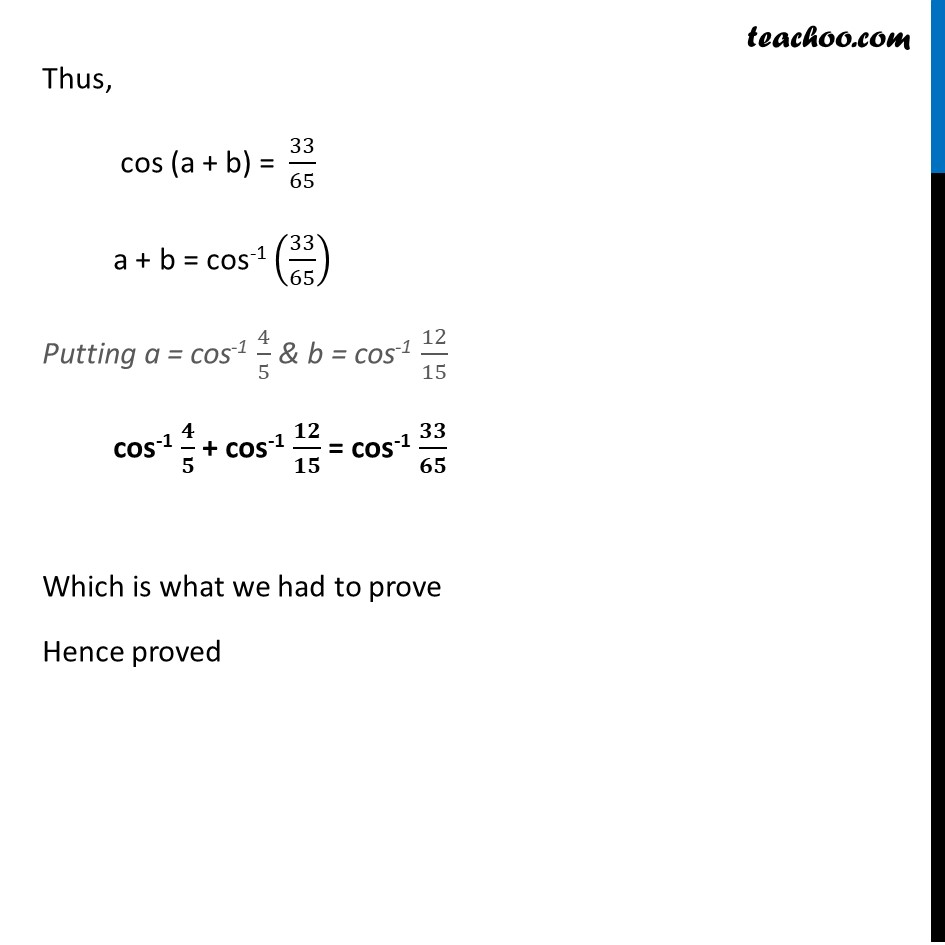Changing of trignometric variables and then applying formula

Chapter 2 Class 12 Inverse Trigonometric Functions
Concept wiseLearn in your speed, with individual attention - Teachoo Maths 1-on-1 Class

### Transcript

Misc 5 Prove cos-1 4/5 + cos-1 12/13 = cos-1 33/65 Let a = cos-1 4/5 and b = cos-1 12/13 Finding sin a, cos a Let a = cos-1 4/5 cos a = 4/5 We know that sin a = √(1 −cos^2⁡𝑎 ) =√(1−(4/5)^2 ) " =" √(9/25) "=" 3/5 Finding sin b, cos b Let b = cos-1 12/13 cos b = 12/13 We know that sin b = √(1 −𝑐𝑜𝑠2 𝑏) = √(1 −(12/13)^2 ) = √(25/169) = 5/13 Now, cos (a + b) = cos a cos b – sin a sin b cos (a + b) = 4/5 × 12/13 – 3/5 × 5/13 = 48/65 − 3/13 = (48 − 3(5))/65 = (48 − 15)/65 = 𝟑𝟑/𝟔𝟓 Putting cos a = 4/5 , sin a = 3/5 & cos b = 12/13 , sin b = 5/13 Thus, cos (a + b) = 33/65 a + b = cos-1 (33/65) Putting a = cos-1 4/5 & b = cos-1 12/15 cos-1 𝟒/𝟓 + cos-1 𝟏𝟐/𝟏𝟓 = cos-1 𝟑𝟑/𝟔𝟓 Which is what we had to prove Hence proved